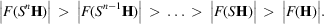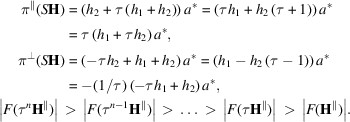International
Tables for
Crystallography
Volume B
Reciprocal space
Edited by U. Shmueli

International Tables for Crystallography (2010). Vol. B, ch. 4.6, pp. 606-607   | 1 | 2 |

## Section 4.6.3.3.1.5. Relationships between structure factors at symmetry-related points of the Fourier image

W. Steurera* and T. Haibacha

aLaboratory of Crystallography, Department of Materials, ETH Hönggerberg, HCI G 511, Wolfgang-Pauli-Strasse 10, CH-8093 Zurich, Switzerland
Correspondence e-mail:  walter.steurer@mat.ethz.ch

#### 4.6.3.3.1.5. Relationships between structure factors at symmetry-related points of the Fourier image

| top | pdf |

The two possible point-symmetry groups in the 1D quasi­periodic case,and, relate the structure factors toA 3D structure with 1D quasiperiodicity results from the stacking of atomic layers with distances following a quasiperiodic sequence. The point groupsdescribing the symmetry of such structures result from the direct productcorresponds to one of the ten crystallographic 2D point groups,can beor. Consequently, 18 3D point groups are possible.

Since 1D quasiperiodic sequences can be described generically as incommensurately modulated structures, their possible point and space groups are equivalent to a subset of thesuperspace groups for IMSs with satellite vectors of the type, i.e., for the quasiperiodic direction  (Janssen et al., 2004).

From the scaling properties of the Fibonacci sequence, some relationships between structure factors can be derived. Scaling the physical-space structure by a factor,, corresponds to a scaling of the perpendicular space by the inverse factor. For the scaling of the corresponding reciprocal subspaces, the inverse factors compared to the direct spaces have to be applied.

The set of vectors r, defining the vertices of a Fibonacci sequence, multiplied by a factor τ coincides with a subset of the vectors defining the vertices of the original sequence (Fig. 4.6.3.10). The residual vertices correspond to a particular decoration of the scaled sequence, i.e. the sequence. The Fourier transform of the sequencethen can be written as the sum of the Fourier transforms of the sequencesand;In terms of structure factors, this can be reformulated asFigure 4.6.3.10 | top | pdf |Part … LSLLSLSL … of a Fibonacci sequencebefore and after scaling by the factor τ. L is mapped onto, S onto. The vertices of the new sequence are a subset of those of the original sequence (the correspondence is indicated by dashed lines). The residual vertices, which give when decoratingthe Fibonacci sequence, form a Fibonacci sequence scaled by a factor.

Hence, phases of structure factors that are related by scaling symmetry can be determined from each other.

Further scaling relationships in reciprocal space exist: scaling a diffraction vectorwith the matrixincreases the magnitudes of structure factors assigned to this particular diffraction vector H,This is due to the shrinking of the perpendicular-space component of the diffraction vector by powers ofwhile expanding the parallel-space component byaccording to the eigenvalues τ andof S acting in the two eigenspacesand:Thus, for scaling n times we obtainwithyielding eventuallyThe scaling of the diffraction vectors H bycorresponds to a hyperbolic rotation (Janner, 1992) with angle, where(Fig. 4.6.3.11):Figure 4.6.3.11 | top | pdf |Scaling operations of the Fibonacci sequence. The scaling operation S acts six times on the diffraction vectoryielding the sequence.

### References

Janner, A. (1992). Decagrammal symmetry of decagonal Al78Mn22 quasicrystal. Acta Cryst. A48, 884–901.
Janssen, T., Janner, A., Looijenga-Vos, A. & de Wolff, P. M. (2004). Incommensurate and commensurate modulated crystal structures. In International Tables for Crystallography, Vol. C, edited by E. Prince, ch. 9.8. Dordrecht: Kluwer Academic Publishers.